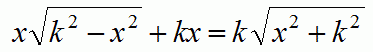Problems

# Equation - 1

For given positive integer k solve the equationIt is known that equation has one root.

#### Input

One positive integer k (k109).

#### Output

Print the single root of the equation with 4 decimal digits.

Time limit 1 second
Memory limit 128 MiB
Input example #1
2

Output example #1
1.5723